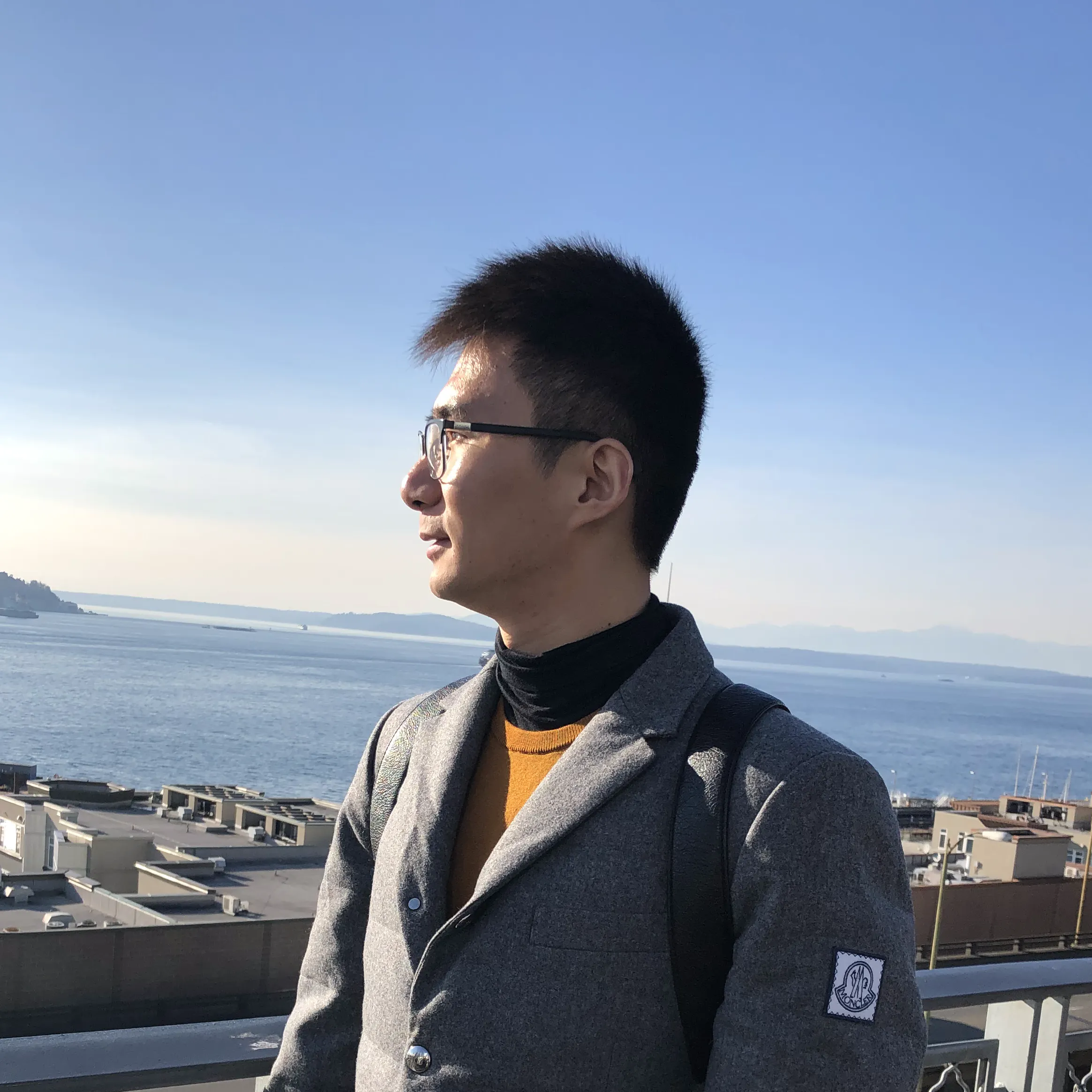# LCD1602MkrUnoShield

This is the customized LCD1602 shield for both Arduino MKR and UNO boards.

BeginnerProtip1 hour1,775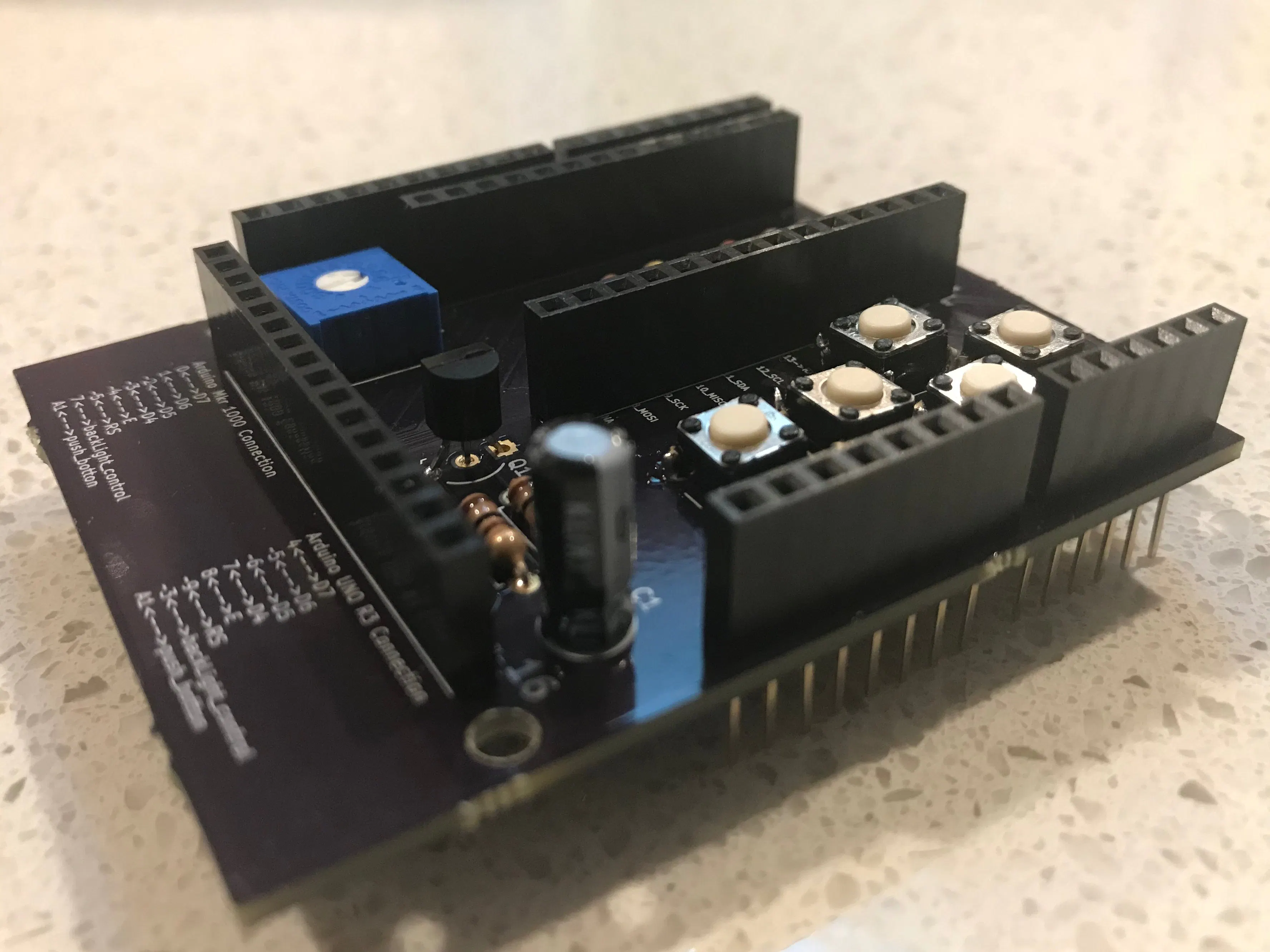## Things used in this project

### Hardware components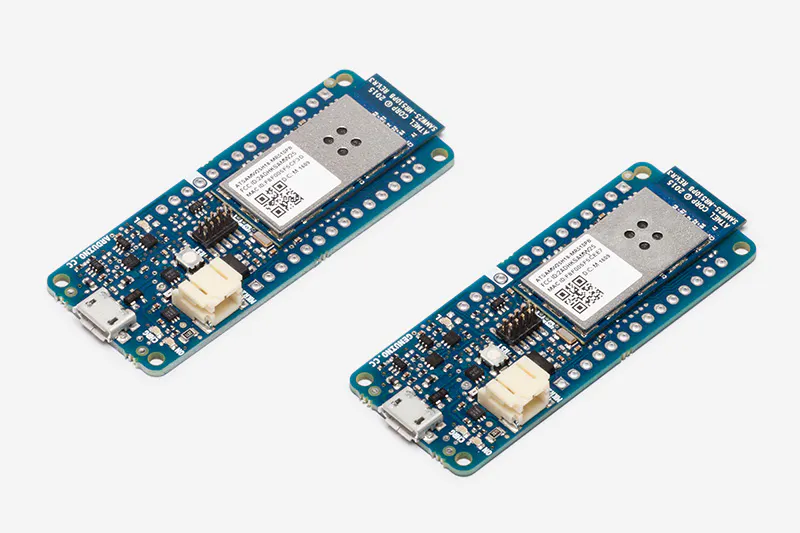Arduino MKR1000
×1Arduino UNO & Genuino UNO
×1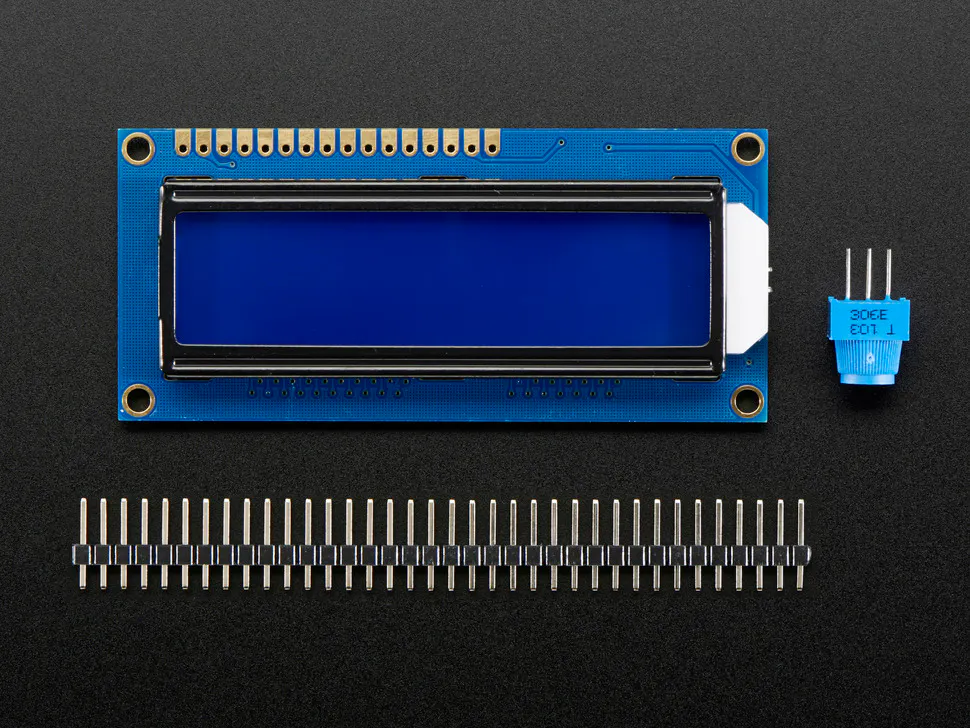Adafruit Standard LCD - 16x2 White on Blue
×1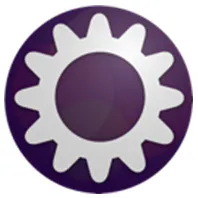OSH Park Custom fabricated PCB
×1

### Software apps and online servicesArduino IDE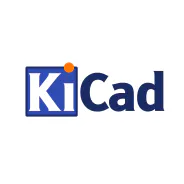## Code

### lcd1602MkrUnoShield_MkrScript

C/C++
This is an example script to use the push buttons to change the screen brightness.
```#define Rbase ((unsigned long)10)
#define Rup ((unsigned long)91)
#define Rleft  ((unsigned long)24)
#define Rright  ((unsigned long)10)
#define Rdown ((unsigned long)4.3)
#define Rselect  ((unsigned long)1)

#include <LiquidCrystal.h>
const int rs = 5, en = 4, d4 = 3, d5 = 2, d6 = 1, d7 = 0;
const int litPin = 7;
LiquidCrystal lcd(rs, en, d4, d5, d6, d7);
int bckLit = 128;
int digit1 = 0;
int digit2 = 0;
int digit3 = 0;
unsigned char key;
int cursorPos = 11;
int sensorValue ;
int KeyTable;
String hint = "max = 255";

void GenerateKeyTable(int vcc,int* array)
{
float resistor;

//////////////1key//////////////////////
resistor = ((float)Rup)/(Rbase + Rup);
*array++ = resistor*vcc;

resistor = ((float)Rleft)/(Rbase + Rleft);
*array++ = resistor*vcc;

resistor = ((float)Rright)/(Rbase + Rright);
*array++ = resistor*vcc;

resistor = ((float)Rdown)/(Rbase + Rdown);
*array++ = resistor*vcc;

resistor = ((float)Rselect)/(Rbase + Rselect);
*array++ = resistor*vcc;

}

unsigned char GetKey(int value)
{
char tmpChar;
unsigned int Rst;

tmpChar = 0;
do{
if(value > KeyTable[tmpChar]) Rst = value - KeyTable[tmpChar];
else  Rst = KeyTable[tmpChar] - value;
tmpChar ++;
}while(Rst > 20);

return tmpChar--;

}

void setup() {
// set up the LCD's number of columns and rows:
lcd.begin(16, 2);

analogWrite(litPin, bckLit);
lcd.print("Welcome to Play");
lcd.setCursor(0,1);
lcd.print("LCDMkrUnoShield");
delay(2000);
lcd.clear();
int tmpInt;
sensorValue  = 1023;
for(tmpInt = 0;tmpInt <31;tmpInt++)
{
Serial.println(KeyTable[tmpInt]);
}

}

void loop() {

analogWrite(litPin, bckLit);
lcd.setCursor(0,1);
lcd.print(hint);
lcd.setCursor(0,0);
lcd.print("Backlight: ");
lcd.print(String(digit3));
lcd.print(String(digit2));
lcd.print(String(digit1));
lcd.setCursor(cursorPos,0);
lcd.cursor();
{
key = GetKey(sensorValue);
// up key
if (key == 1){
if (cursorPos == 11){
digit3++;
if (digit3 >=3){
digit3=0;
}
}
if (cursorPos == 12) {
digit2++;
if (digit2 >=10){
digit2=0;
}
}
if (cursorPos == 13) {
digit1++;
if (digit1 >=10){
digit1=0;
}
}
}

// down key
if (key == 4){
if (cursorPos == 11){
digit3--;
if (digit3 <0){
digit3=2;
}
}
if (cursorPos == 12) {
digit2--;
if (digit2 <0){
digit2=9;
}
}
if (cursorPos == 13) {
digit1--;
if (digit1 <0){
digit1=9;
}
}
}

// left key
if (key == 2){
cursorPos--;
if (cursorPos <=11){
cursorPos = 11;
}
}

// right key
if (key == 3) {
cursorPos++;
if (cursorPos >=13){
cursorPos = 13;
}
}

// select key
if (key==5){
bckLit = digit3 * 100 + digit2 * 10 + digit1;
if (bckLit >= 255){
bckLit = 255;
digit3 = 2;
digit2 = 5;
digit1 = 5;
}
}
}
delay(150);
}
```

### lcd1602MkrUnoShield_UnoScript

C/C++
This is an example script to use Arduino UNO board to change the LD screen brightness
```#define Rbase ((unsigned long)10)
#define Rup ((unsigned long)91)
#define Rleft  ((unsigned long)24)
#define Rright  ((unsigned long)10)
#define Rdown ((unsigned long)4.3)
#define Rselect  ((unsigned long)1)

#include <LiquidCrystal.h>
const int rs = 9, en = 8, d4 = 7, d5 = 6, d6 = 5, d7 = 4;
const int litPin = 3;
LiquidCrystal lcd(rs, en, d4, d5, d6, d7);
int bckLit = 128;
int digit1 = 0;
int digit2 = 0;
int digit3 = 0;
unsigned char key;
int cursorPos = 11;
int sensorValue ;
int KeyTable;
String hint = "max = 255";

void GenerateKeyTable(int vcc,int* array)
{
float resistor;

//////////////1key//////////////////////
resistor = ((float)Rup)/(Rbase + Rup);
*array++ = resistor*vcc;

resistor = ((float)Rleft)/(Rbase + Rleft);
*array++ = resistor*vcc;

resistor = ((float)Rright)/(Rbase + Rright);
*array++ = resistor*vcc;

resistor = ((float)Rdown)/(Rbase + Rdown);
*array++ = resistor*vcc;

resistor = ((float)Rselect)/(Rbase + Rselect);
*array++ = resistor*vcc;

}

unsigned char GetKey(int value)
{
char tmpChar;
unsigned int Rst;

tmpChar = 0;
do{
if(value > KeyTable[tmpChar]) Rst = value - KeyTable[tmpChar];
else  Rst = KeyTable[tmpChar] - value;
tmpChar ++;
}while(Rst > 10);

return tmpChar--;

}

void setup() {
// set up the LCD's number of columns and rows:
lcd.begin(16, 2);

analogWrite(litPin, bckLit);
lcd.print("Welcome to Play");
lcd.setCursor(0,1);
lcd.print("LCDMkrUnoShield");
delay(2000);
lcd.clear();
int tmpInt;
sensorValue  = 1023;
for(tmpInt = 0;tmpInt <31;tmpInt++)
{
Serial.println(KeyTable[tmpInt]);
}

}

void loop() {

analogWrite(litPin, bckLit);
lcd.setCursor(0,1);
lcd.print(hint);
lcd.setCursor(0,0);
lcd.print("Backlight: ");
lcd.print(String(digit3));
lcd.print(String(digit2));
lcd.print(String(digit1));
lcd.setCursor(cursorPos,0);
lcd.cursor();
{
key = GetKey(sensorValue);
// up key
if (key == 1){
if (cursorPos == 11){
digit3++;
if (digit3 >=3){
digit3=0;
}
}
if (cursorPos == 12) {
digit2++;
if (digit2 >=10){
digit2=0;
}
}
if (cursorPos == 13) {
digit1++;
if (digit1 >=10){
digit1=0;
}
}
}

// down key
if (key == 4){
if (cursorPos == 11){
digit3--;
if (digit3 <0){
digit3=2;
}
}
if (cursorPos == 12) {
digit2--;
if (digit2 <0){
digit2=9;
}
}
if (cursorPos == 13) {
digit1--;
if (digit1 <0){
digit1=9;
}
}
}

// left key
if (key == 2){
cursorPos--;
if (cursorPos <=11){
cursorPos = 11;
}
}

// right key
if (key == 3) {
cursorPos++;
if (cursorPos >=13){
cursorPos = 13;
}
}

// select key
if (key==5){
bckLit = digit3 * 100 + digit2 * 10 + digit1;
if (bckLit >= 255){
bckLit = 255;
digit3 = 2;
digit2 = 5;
digit1 = 5;
}
}
}
delay(150);
}
```

## Credits

### zhaoshentech

6 projects • 57 followers
An enthusiastic for startups!